TitleCollege Algebra
Tutorial 58: ProbabilityLearning Objectives

 After completing this tutorial, you should be able to: Find the probability of an empirical event. Find a theoretical probability. Know when two events are mutually exclusive. Use basic properties of probability to find various probabilities. Know when two events are independent of each other. Know how to find the probability of the intersection of two independent events.Introduction

 In this tutorial we will be going over probability. This will include learning some of the terminology that goes with the different aspects of probability.  Things like experiment, sample space and event to name a few.  A lot of times people associate probability with gambling, like playing cards and lotto.  It can be used to find out your chances of winning :-) or losing :-( a game of chance.  But it can also be used in other areas like research and advertising.  A doctor might want to weigh the  risks of putting a patient on a new drug and may look at the probability of their chances of success on the drug.  A company may take a survey of people on certain products to see what is the probability that they will pick their product.  So probability can be fun and games, but it can also be useful in a professional setting.  I think you are ready to venture into the world of probability.Tutorial

 Experiment

 An experiment is an act for which the outcome is uncertain. Examples of experiments are rolling a die, tossing a coin, surveying a group of people on their favorite soft drink, etc...

 Sample Space

 A sample space S for an experiment is the set of all possible outcomes of the experiment such that each outcome corresponds to exactly one element in S.  The elements of S are called sample points.  If there is a finite number of sample points, that number is denoted n(S), and S is said to be a finite sample space. For example, if our experiment is rolling a single die, the sample space would be S = {1, 2, 3, 4, 5, 6}. If our experiment is tossing a single coin, our sample space would be S = {Heads, Tails}.  If our experiment is surveying a group of people on their favorite soft drink, our sample space would be all of the soft drinks on the survey.

 Event

 Any subset E of a sample space for an experiment is called an event for that experiment. For example, if our experiment is rolling a single die, an event E could be rolling an even number, thus E = {2, 4, 6}.  If our experiment is tossing a single coin, an event E could be tossing a Tail, where E = {Tails}.  If our experiment is surveying a group of people on their favorite soft drink, an event E could be picking a diet soft drink.

 Empirical Probability

 Finding the probability of an empirical event is specifically based on direct observations or experiences. For example, a survey may have been taken by a group of people.  If the data collected is used to find the probability of an event tied to the survey, it would be an empirical probability.   Or if a scientist did research on a topic and recorded the outcome and the data from this is used to find the probability of an event tied to the research, it would also be an empirical probability.

 Empirical Probability Formula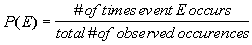P(E) represents the probability that an event, E, will occur. The numerator of this probability is the number of times or ways that specific event occurs. The denominator of this probability is the overall number of ways that the experiment itself could occur.Example 1:   The table below lists the results of a student survey pertaining to favorite ethnic foods.  Each student chose only one type of ethnic food for the survey.

 Type Italian Chinese Japanese Thai Mexican Other Number 15 20 3 4 30 10

Using the given table find the probability that a) a student's favorite ethnic food is Chinese, and b) a student's favorite ethnic food is Mexican.  Round answers to three decimal places.

 1a) a student's favorite ethnic food is Chinese:

 To find this probability, we need to know n(E) = n(students whose favorite ethnic food is Chinese), which is the number of students who preferred Chinese food and n(S) = n(students surveyed), which is the total number of students surveyed. What do you think n(E) = n(students whose favorite ethnic food is Chinese) is?  If you said 20 you are correct.  20 students indicated that their favorite ethnic food is Chinese. What do you think n(S) = n(students surveyed) is?  If you said 82 give yourself a pat on the back.  If you total 15 + 20 + 3 + 4 + 30 + 10 you get 82. Putting this together in the Empirical Probability formula you get: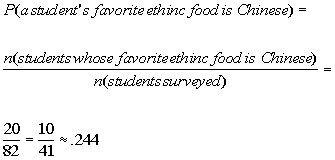*putting in the numbers found above *reducing the fraction  *rounding to 3 decimal places

 The probability that a student's favorite ethnic food is Chinese is .244.

 1b) a student's favorite ethnic food is Mexican:

 To find this probability, we need to know n(E) = n(students whose favorite ethnic food is Mexican), which is the number of students who preferred Mexican food and n(S) = n(students surveyed), which is the total number of students surveyed. What do you think n(E) = n(students whose favorite ethnic food is Mexican) is?  If you said 30 you are correct.  30 students indicated that their favorite ethnic food is Mexican.   What do you think n(S) = n(students surveyed) is?  If you said 82 give yourself a pat on the back.  As found in example 1a above, the total surveyed is 82. Putting this together in the Empirical Probability formula you get: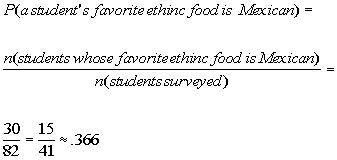*putting in the numbers found above *reducing the fraction  *rounding to 3 decimal places

 The probability that a student's favorite ethnic food is Mexican is .366.

 Equiprobable Space

A sample space S is called an equiprobable space if and only if all the simple events are equally likely to occur.

Some quick examples of this are:

 A toss of a fair coin.  It is equally likely for a head to show up as it is for a tail.  Select a name at random from a hat.  Since it is at random, each name is equally likely to be picked.  Throwing a well balanced die.  Each number on the die has the same amount of chance of coming up.

 Theoretical Probability

 Theoretical probability is finding the probability of events that come from an equiprobable sample space or, in other words, a sample space of known equally likely outcomes. For example, finding various probabilities dealing with the roll of a die, a toss of a coin, or a picking of a name from a hat.

 Theoretical Probability FormulaIf E is an event of sample space S, where n(E) is the number of equally likely outcomes of event E and n(S) is the number of equally outcomes of sample space S, then the probability of event E occurring can be found using the Theoretical Probability Formula above.

 Mutually Exclusive

 In general, events E and F are said to be mutually exclusive if and only if  they have no elements in common. For example, if the sample space is rolling a die, where S = {1, 2, 3, 4, 5, 6}, and E is the event of rolling an even number, E = {2, 4, 6} and F is the event of rolling an odd number, F = {1, 3, 5}, E and F are mutually exclusive, because they have NO elements in common.  Now lets say that event G is rolling a number less than 4, G = {1, 2, 3}.  Would event G and E be mutually exclusive?  If you said no, you are correct, they have one element, the number 2, in common. G and F would not be mutually exclusive either.

 Properties of Probability

 Property 1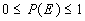It stands to reason that if the bottom number of the probability is the total number - which is the highest number  - than the probability will never exceed 1.

 Property 2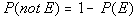Either an element is in E or it is not in E.  So if, P(E) = 1/4 then P(not E) = 3/4.

 Property 3This is just like Property 2 in reverse.

 Property 4 "Or" probabilities with  mutually exclusive events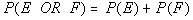Since we are dealing with sets that are mutually exclusive, this means they have no elements in common.  So we can just add the two probabilities together without running a risk of having something counted twice.

 Property 5 "Or" probabilities with events that are NOT mutually exclusive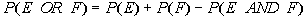Since we are dealing with events that are NOT mutually exclusive, we run the risk of elements being counted twice if we just add them together as in Property 4 above.  You need to subtract the intersection to get rid of the elements that were counted twice.  In other words,  you may have some elements in common, so if we add the number of elements in E to the number of elements of  F, we may be adding some elements twice, so to avoid this we need to subtract the number of elements in the intersection of the two events - which would be all the elements that are in both sets.Example 2:   A pair of fair dice is tossed.  Determine the probability that a) at least one of the dice shows a 6 and b) the sum of the two numbers is 5.  Round answers to three decimal places.

Here is a table of all the possible outcomes of having a pair of dice tossed:

 (1, 1) (1, 2) (1, 3) (1, 4) (1, 5) (1, 6) (2, 1) (2, 2) (2, 3) (2, 4) (2, 5) (2, 6) (3, 1) (3, 2) (3, 3) (3, 4) (3, 5) (3, 6) (4, 1) (4, 2) (4, 3) (4, 4) (4, 5) (4, 6) (5, 1) (5, 2) (5, 3) (5, 4) (5, 5) (5, 6) (6, 1) (6, 2) (6, 3) (6, 4) (6, 5) (6, 6)

 2a) at least one of the dice shows a 6:

 To find this probability we need to know n(E) = n(at least one of the dice shows a 6), which is the number of rolls of two dice that have at least one 6 showing and n(S) = n(rolls of a pair of fair dice), which is the total number of ways a pair of fair dice can be rolled. What do you think n(E) = n(at least one of the dice shows a 6) is?  If you said 11 you are correct.  Looking at the table above, there are 11 rolls of two dice that contain at least one 6.   What do you think n(S) = n(rolls of a pair of fair dice) is?  If you said 36 give yourself a pat on the back.  Looking at the table above, there are 36 possible rolls of two fair dice.   Putting this together in the Theoretical Probability formula you get: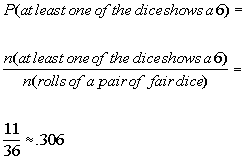*putting in the numbers found above *rounding to 3 decimal places

 The probability that at least one of the dice shows a 6 is .306.

 2b) the sum of the two numbers is 5:

 To find this probability we need to know n(E) = n(the sum of the two numbers is 5), which is the number of rolls of two dice that have a sum of 5 and n(S) = n(rolls of a pair of fair dice), which is the total number of ways a pair of fair dice can be rolled. What do you think n(E) = n(the sum of the two numbers is 5) is?  If you said 4 you are correct.  Looking at the table above, 4 rolls of two die {(4, 1), (3, 2), (2, 3), (1, 4)} have a sum of 5.   What do you think n(S) = n(rolls of a pair of fair dice) is?  If you said 36 give yourself a pat on the back.  Looking at the table above, there are 36 possible rolls of two fair dice.   Putting this together in the Theoretical Probability formula you get: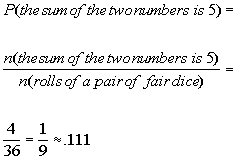*putting in the numbers found above *reducing the fraction  *rounding to 3 decimal places

 The probability that the sum of the two numbers is 5 is .111.Example 3:   From a group of 10 women and 5 men, 2 people are selected at random to form a committee.  Find the probability that a) only men are selected and b) exactly 1 man and 1 woman is selected. Round answers to three decimal places.

 3a) only men are selected:

 To find this probability we need to know n(E) = n(only men are selected), which is the number of committees that contain 2 men and n(S) = n(2 person committees), which is the total number of 2 person committees. What do you think n(E) = n(only men are selected) is?  Since we are counting committees that means we are counting groups of people, which means we need to use combinations to count them.  If you need a review on combinations, feel free to go to Tutorial 57: Combinations. If you said 10 you are correct.  Let's see how we get that number. Here is what the committee looks like:  we would have to have 2 men to meet this condition:  2 MEN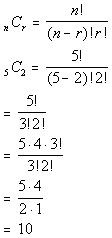*Comb. of n = 5 men taken r = 2 at a time *Eval. inside ( ) *Expand 5! until it gets to 3!   which is the larger ! in the den. *Cancel out 3!'s

 As mentioned above, n(E) = n(only men are selected) = 10. If you have a factorial key, you can put it in as 5!, divided by 3!, divided by 2! and then press enter or =.  If you don't have a factorial key, you can simplify it as shown above and then enter it in.

 What do you think n(S) = n(2 person committees) is?  If you said 105 give yourself a pat on the back.  Again you would have to use combinations to figure this one out.  Overall, there are 10 women and 5 men for a total of 15 people, taken 2 at a time.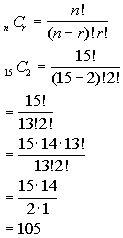*Comb. of n = 15 people taken r = 2 at a time *Eval. inside ( ) *Expand 15! until it gets to 13!   which is the larger ! in the den. *Cancel out 13!'s

 As mentioned above, n(S) = n(2 person committees) = 105. If you have a factorial key, you can put it in as 15!, divided by 13!, divided by 2! and then press enter or =.  If you don't have a factorial key, you can simplify it as shown above and then enter it in.    Putting this together in the Theoretical Probability formula you get: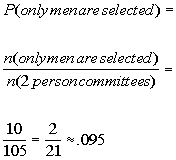*putting in the numbers found above *reducing the fraction  *rounding to 3 decimal places

 The probability that only men are selected is .095.

 3b) exactly 1 man and 1 woman:

 To find this probability we need to know n(E) = n(1 man and 1 woman are selected), which is the number of committees that contain 1 man and 1 woman and n(S) = n(2 person committees), which is the total number of 2 person committees. What do you think n(E) = n(1 man and 1 woman are selected) is?  Since we are counting committees that means we are counting groups of people, which means we need to use combinations to count them.  If you need a review on combinations, feel free to go to Tutorial 57: Combinations. If you said 50 you are correct.  Let's see how we get that number. Here is what the committee looks like:  we would have to have 1 man and 1 woman to meet this condition:  1 MAN  1 WOMAN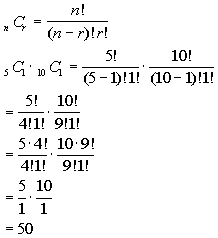*Comb. of n = 5 men taken r = 1 at a time *Comb. of n = 10 women taken r = 1 at a time *Eval. inside ( ) *Expand 5! until it gets to 4! and 10! until it gets to 9!   which are the larger !'s in the den. *Cancel out 4!'s and 9!'s

 As mentioned above, n(E) = n(1 man and 1 woman selected) = 50. If you have a factorial key, you can put it in as 5!, divided by 4!, divided by 1! times 10!, divided by 9!, divided by 1! and then press enter or =.  If you don't have a factorial key, you can simplify it as shown above and then enter it in.

 What do you think n(S) = n(2 person committees) is?  If you said 105 give yourself a pat on the back. As found in example 3a above, the total number of 2 person committees is 105.

 Putting this together in the Theoretical Probability formula you get: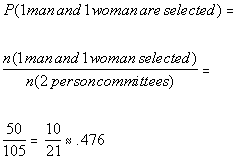*putting in the numbers found above *reducing the fraction  *rounding to 3 decimal places

 The probability that exactly one man and one woman are selected is .476.Example 4:   One student's name will be picked at random to win a CD player.  There are 12 male seniors, 15 female seniors, 10 male juniors, 5 female juniors, 2 male sophomores, 4 female sophomores, 11 male freshmen and 12 female freshman.  Find the probability that  a) a senior or a junior is picked, b) a freshman or a female is picked, and c) a freshman is NOT picked.   Round answers to three decimal places.

 4a) a senior or a junior is picked:

Note that these two events are mutually exclusive.  You CANNOT be a senior and a junior at the same time.  So we can use the formula (property 4)Let's break it down first by finding each separate probability.

P(a senior is picked)

 To find this probability we need to know n(E) = n(seniors), which is the number of seniors and n(S) = n(students), which is the total number of students. What do you think n(E) = n(seniors) is?  If you said 27 you are correct.  There are 12 male seniors and 15 female seniors for a total of 27 seniors.   What do you think n(S) = n(students) is?  If you said 71 give yourself a pat on the back.  If we add all the students together we get 71.   Putting this together in the Theoretical Probability formula you get: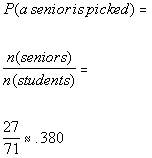*putting in the numbers found above *rounding to 3 decimal places

P(a junior is picked)

 To find this probability we need to know n(E) = n(juniors), which is the number of juniors and n(S) = n(students), which is the total number of students. What do you think n(E) = n(juniors) is?  If you said 15 you are correct.  There are 10 male juniors and 5 female juniors for a total of 15 juniors.   What do you think n(S) = n(students) is?  If you said 71 give yourself a pat on the back.  If we add all the students together we get 71.   Putting this together in the Theoretical Probability formula you get: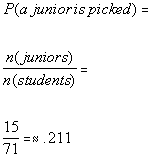*putting in the numbers found above *rounding to 3 decimal places

Putting the two probabilities of mutually exclusive events together we get: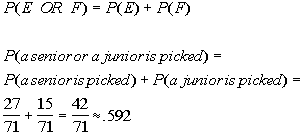*putting in the numbers found above *rounding to 3 decimal places

 The probability that a senior or a junior is picked is .592.

 4b) a freshman or a female is picked:

Note that these two events are NOT mutually exclusive.  You CAN be a freshman and a female at the same time.  So we can use the formula (property 5)Let's break it down first by finding each separate probability.

P(a freshman is picked)

 To find this probability we need to know n(E) = n(freshmen), which is the number of freshmen and n(S) = n(students), which is the total number of students. What do you think n(E) = n(freshmen) is?  If you said 23 you are correct.  There are 11 male freshmen and 12 female freshmen for a total of 23 freshmen.   What do you think n(S) = n(students) is?  If you said 71 give yourself a pat on the back.  If we add all the students together we get 71.   Putting this together in the Theoretical Probability formula you get: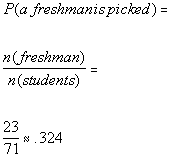*putting in the numbers found above *rounding to 3 decimal places

P(a female is picked)

 To find this probability we need to know n(E) = n(females), which is the number of females and n(S) = n(students), which is the total number of students. What do you think n(E) = n(females) is?  If you said 36 you are correct.  There are 15 female seniors, 5 female juniors, 4 female sophomores and 12 female freshman for a total of 36 females.   What do you think n(S) = n(students) is?  If you said 71 give yourself a pat on the back.  If we add all the students together we get 71.   Putting this together in the Theoretical Probability formula you get: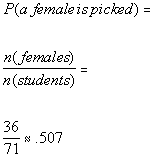*putting in the numbers found above *rounding to 3 decimal places

P(a freshman AND female is picked)

 To find this probability we need to know n(E) = n(freshman AND female), which is the number of female freshmen and n(S) = n(students), which is the total number of students. What do you think n(E) = n(freshmen AND female) is?  If you said 12 you are correct.  There are 12 female freshmen.   What do you think n(S) = n(students) is?  If you said 71 give yourself a pat on the back.  If we add all the students together we get 71.   Putting this together in the Theoretical Probability formula you get:*putting in the numbers found above *rounding to 3 decimal places

Putting the probabilities of non mutually exclusive events together we get: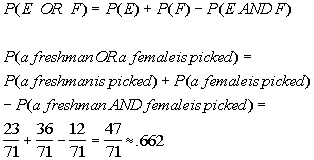*putting in the numbers found above *rounding to 3 decimal places

 The probability that a freshman or a female is picked is .662.

 4c) a freshman is NOT picked:

One way we can approach this is to use Property 2To find this probability we need to know n(E) = n(freshmen), which is the number of freshman and n(S) = n(students), which is the total number of students. What do you think n(E) = n(freshmen) is?  If you said 23 you are correct.  There are 11 male freshmen and 12 female freshmen for a total of 23 freshman.   What do you think n(S) = n(students) is?  If you said 71 give yourself a pat on the back.  If we add all the students together we get 71.   Putting this together in the Theoretical Probability formula you get:*putting in the numbers found above *rounding to 3 decimal places

 So, the probability a student picked is NOT a freshman is*putting in the numbers found above *rounding to 3 decimal places

 The probability that a freshman is NOT picked is .676.

 Independent Events E and F are Independent Events if an only if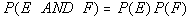Two events are independent of each other if the outcome of one event does not affect the outcome of the other event. You can use this formula to show if two events are independent or not.Example 5:   If two cards are drawn from a deck of cards, WITH REPLACEMENT, find the probability that the 1st card is a heart AND the 2nd card is an ace.   Round answer to three decimal places.

 Since it said WITH REPLACEMENT, that means the outcome of the 1st card does NOT affect the outcome of the 2nd card, which means these two events are independent.  So we can use the formulato find our probability. Let's break it down.

P(1st card is a heart)

 To find this probability we need to know n(E) = n(hearts), which is the number of hearts in a deck of cards and n(S) = n(cards), which is the total number of cards in a deck. What do you think n(E) = n(hearts) is?  If you said 13 you are correct. There are 13 cards in a deck that have heart on them.   What do you think n(S) = n(cards) is?  If you said 52 give yourself a pat on the back. There are 52 cards in a deck. Putting this together in the Theoretical Probability formula you get: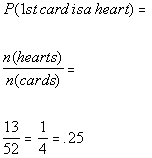*putting in the numbers found above *reducing the fraction  *rounding to 3 decimal places

P(2nd card is an ace)

 To find this probability we need to know n(E) = n(aces), which is the number of aces in a deck of cards and n(S) = n(cards), which is the total number of cards in a deck. What do you think n(E) = n(aces) is?  If you said 4 you are correct. There are 4 cards in a deck that have an ace on them.   What do you think n(S) = n(cards) is?  If you said 52 give yourself a pat on the back. There are 52 cards in a deck. Putting this together in the Theoretical Probability formula you get: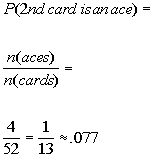*putting in the numbers found above *reducing the fraction  *rounding to 3 decimal places

Putting the probabilities of two events that are independent together we get:*putting in the numbers found above *rounding to 3 decimal placesPractice Problems

 These are practice problems to help bring you to the next level.  It will allow you to check and see if you have an understanding of these types of problems. Math works just like anything else, if you want to get good at it, then you need to practice it.  Even the best athletes and musicians had help along the way and lots of practice, practice, practice, to get good at their sport or instrument.  In fact there is no such thing as too much practice. To get the most out of these, you should work the problem out on your own and then check your answer by clicking on the link for the answer/discussion for that  problem.  At the link you will find the answer as well as any steps that went into finding that answer.Practice Problems 1a - 1b:

The table below lists the results of a student survey pertaining to favorite types of music.

 Music Rock Jazz Country Classical Rap Number 51 24 33 5 15

Using the given table find the probability that

 1a. a student's favorite type of music is jazz.  Round answer to three decimal places. (answer/discussion to 1a)

 1b. a student's favorite type of music is classical.   Round answer to three decimal places. (answer/discussion to 1b)Practice Problems 2a - 2e: You are dealt one card.  Find the probability that you are dealt

 2a. a jack.  Round answer to three decimal places. (answer/discussion to 2a)

 2b. a heart or club.  Round answer to three decimal places. (answer/discussion to 2b)

 2c. a heart and a club.  Round answer to three decimal places. (answer/discussion to 2c)Practice Problems 3a - 3b: From a group of 7 freshmen, 9 sophomores, 8 juniors, and 10 seniors, 6 names will be drawn.  Find the probability that

 3a. 2 freshmen, 2 sophomores and 2 juniors are selected.  Round answer to three decimal places. (answer/discussion to 3a)

 3b. only seniors are selected.  Round answer to three decimal places. (answer/discussion to 3b)Practice Problems 4a - 4b: You roll a single die two times.  Find the probability that

 4a. a six is rolled the 1st time and an odd number is rolled a 2nd time.  Round answer to three decimal places. (answer/discussion to 4a)

 4b. a number less than or equal to 2 is rolled the 1st time and an even number is rolled the 2nd time.  Round answer to three decimal places. (answer/discussion to 4b)Need Extra Help on these Topics?

The following are webpages that can assist you in the topics that were covered on this page:

 http://www.mathleague.com/help/percent/percent.htm#whatisanevent This webpage goes over events.  http://www.mathleague.com/help/percent/percent.htm#probability This webpage goes over probability.

Go to Get Help Outside the Classroom found in Tutorial 1: How to Succeed in a Math Class for some more suggestions.

Last revised on May 21, 2011 by Kim Seward.